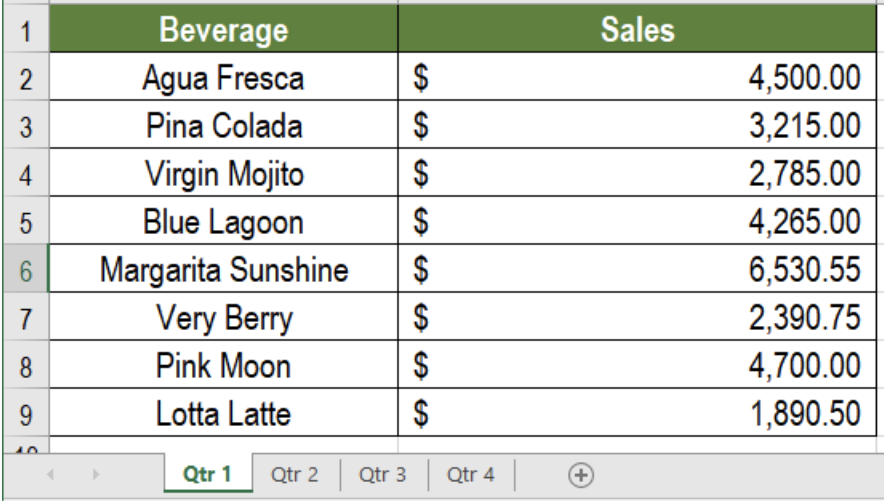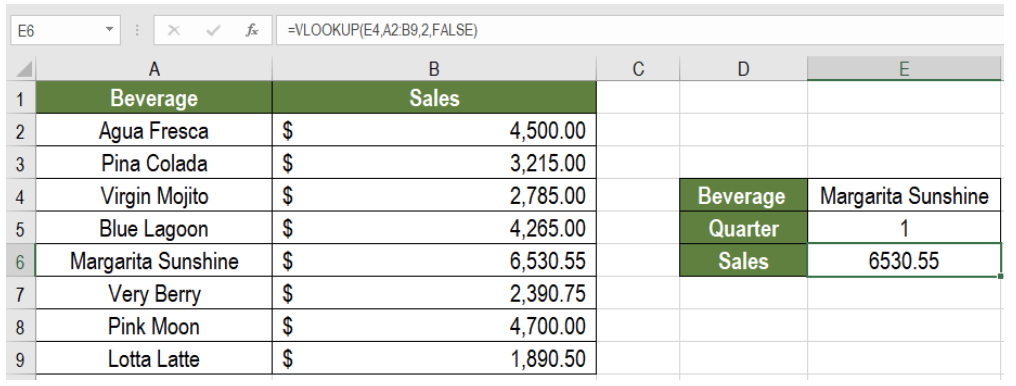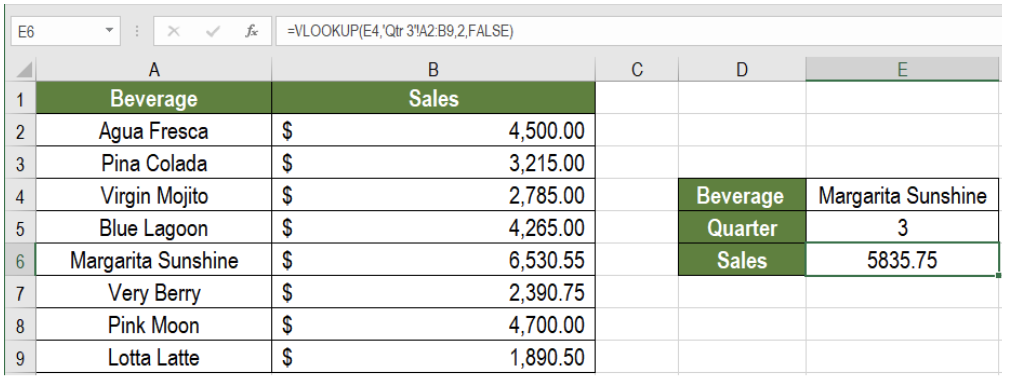Get instant live expert help with Excel or Google Sheets“My Excelchat expert helped me in less than 20 minutes, saving me what would have been 5 hours of work!”

#### Post your problem and you’ll get Expert help in seconds.

Your message must be at least 40 characters
Our professional Expert are available now. Your privacy is guaranteed.

# How to Apply a VLOOKUP Between WorksheetsFigure 1. Sample Data set for VLOOKUP between Worksheets

VLOOKUP is one of the most used formulas in Excel. In its generic form VLOOKUP can only refer data from the same worksheet. To apply a VLOOKUP between worksheets, we must reference the other worksheet(s) in the VLOOKUP formula. In this tutorial, we will learn how to apply a VLOOKUP between worksheets.

## The Generic formula for VLOOKUP

`=VLOOKUP(lookup_value,range,column_number,match_type)`

This formula allows VLOOKUP to lookup data from the same sheet. The following example uses a beverage sales dataset. It has the columns for the beverage name and prices in two worksheets.

To retrieve the sales for Margarita Sunshine in quarter 1, we use the formula `=VLOOKUP(E4,A2:B9,2,FALSE)` in cell E6. This will return the sales for Margarita Sunshine in the first quarter.Figure 2. VLOOKUP Within Same worksheet

However, we would not be able to return the sales for Margarita Sunshine in quarter 3 using this formula. To do that, we would need to change the formula.

## The Generic formula for VLOOKUP Between Worksheets

`=VLOOKUP(lookup_value, sheet!range,column_number,match_type)`

The only difference in this formula is that it includes the sheet name of the worksheet. This lets us apply VLOOKUP between two sheets. The reference of the second sheet as the lookup_value lets us look for the value in the other sheet. When VLOOKUP finds the value, the corresponding value is returned. The match_type is generally assigned as false to find an exact match.

## Example

To return the sales for Margarita Sunshine in quarter 3 from the previous example, we need to:

• Go to cell E6.
• Apply the formula `=VLOOKUP(E4,'Qtr 3'!A2:B9,2,FALSE) `to cell E6.
• Press Enter.Figure 3. How to Apply VLOOKUP between Worksheets

This will help apply VLOOKUP between the two worksheets. It will return the  sales for Margarita Sunshine in the third quarter.

## Notes

We need to ensure the lookup_value exists in the second sheet. Otherwise, Excel will generate an #N/A error.

Most of the time, the problem we will need to solve will be more complex than a simple application of a formula or function. If you want to save hours of research and frustration, try our live Excelchat service! Our Excel Experts are available 24/7 to answer any Excel question you may have. We guarantee a connection within 30 seconds and a customized solution within 20 minutes.

### Did this post not answer your question? Get a solution from connecting with the expert.Another blog reader asked this question today on Excelchat:## Subscribe to Excelchat.coAnother blog reader asked this question today on Excelchat: